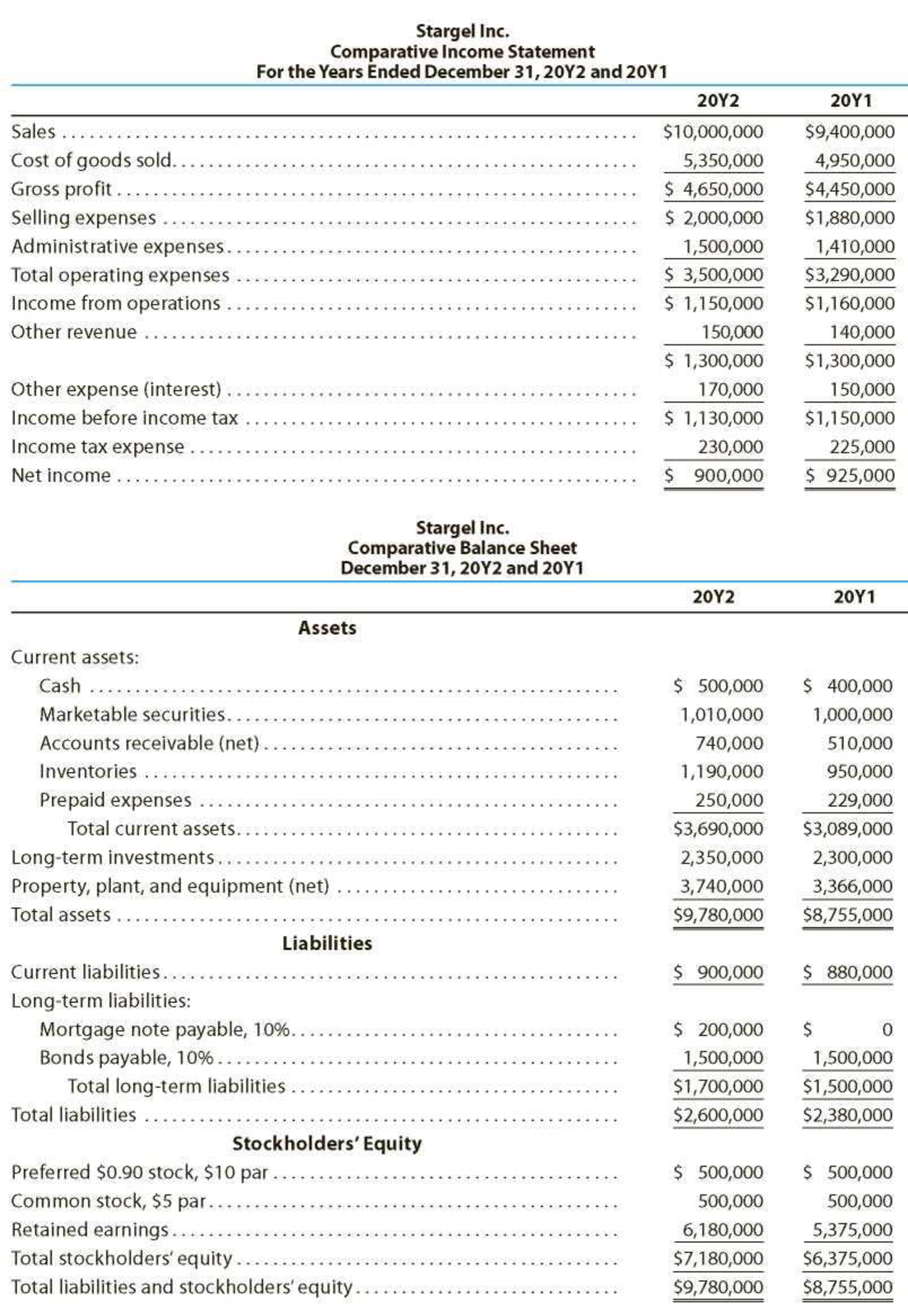Chapter 17, Problem 4PB

Chapter
Section
Textbook Problem
1 views

# Measures of liquidity, solvency and profitabilityThe comparative financial statements of Stargel Inc. are as follows. The market price of Stargel common stock was $11970 on December 31, 20Y2.InstructionsDetermine the following measures for 20Y2, rounding to one decimal place including percentages, except for per-share amounts: 1. Working capital 2. Current ratio 3. Quick ratio 4. Accounts receivable turnover 5. Number of days’ sales in receivables 6. Inventory turnover 7. Number of days’ sales in inventory 8. Ratio of fixed assets to long-term liabilities 9. Ratio of liabilities to stockholders’ equity 10. Times interest earned 11. Asset turnover 12. Return on total assets 13. Return on stockholders’ equity 14. Return on common stockholders’ equity 15. Earnings per share on common stock 16. Price-earnings ratio 17. Dividends per share of common stock 18. Dividend yield To determine Calculate the following ratios measures for 20Y2. 1. 1. Working capital 2. 2. Current ratio 3. 3. Quick ratio 4. 4. Accounts receivable turnover 5. 5. Number of days' sales in receivables 6. 6. Inventory turnover ratio 7. 7. Number of days' sales in inventory 8. 8. Ratio of fixed assets to long-term liabilities 9. 9. Ratio of liabilities to stockholders’ equity 10. 10. Times interest earned ratio 11. 11. Asset turnover ratio 12. 12. Return on total assets 13. 13. Return on stockholders' equity 14. 14. Return on common stockholders' equity 15. 15. Earnings per share on common stock 16. 16. Price earnings ratio 17. 17. Dividends per share of common stock 18. 18. Dividend yield Explanation Financial Ratios: Financial ratios are the metrics used to evaluate the capabilities, profitability, and overall performance of a company. 1. Working capital Working capital = Current assets – Current liabilities =$3,690,000 – $900,000=$2,790,000

Description:

Working capital is determined as the difference between current assets and current liabilities.

Formula:

Working capital = Current assets – Current liabilities

2. Current ratio

Current ratio=Current assetsCurrentliabilities=$3,690,000$900,000=4.1

Description:

Current ratio is used to determine the relationship between current assets and current liabilities. The ideal current ratio is 2:1.  Current assets include cash and cash equivalents, short-term investments, net, accounts and notes receivables, net, inventories, and prepaid expenses and other current assets. Current liabilities include short-term obligations and accounts payable.

Formula:

Current ratio=Current assetsCurrentliabilities

3. Quick ratio

Quick ratio =Quick assets Currentliabilities=$2,250,000$900,000=2.5

Description:

Acid-Test Ratio is the ratio denotes that this ratio is a more rigorous test of solvency than the current ratio. It is determined by dividing quick assets and current liabilities. The acceptable acid-test ratio is 0.90 to 1.00. It is referred as quick ratio. Use the following formula to determine the acid-test ratio:

Acid Ratio=Quick assetsCurrentliabilities

4. Accounts receivable turnover

Accounts receivables turnover ratio}=Net credit salesAverage accounts receivables=$10,000,000$625,000=16.0

Description:

Accounts receivables turnover ratio is mainly used to evaluate the collection process efficiency. It helps the company to know the number of times the accounts receivable is collected in a particular time period. Main purpose of accounts receivable turnover ratio is to manage the working capital of the company. This ratio is determined by dividing credit sales and sales return.

Formula:

Accounts receivables turnover ratio}=Net credit salesAverage accounts receivables

Average accounts receivable is determined as follows:

Average accounts receivables }(Opening accounts receivables + Closing accounts receivables )2=$740,000+$510,0002=$625,000 5. Number of days’ sales in receivables Number of days’ sales in receivable }=Average accounts receivable Average daily sales=$625,00027,397.26=22.8days

Description:

Number of days’ sales in receivables is used to determine the number of days a particular company takes to collect accounts receivables.

Formula:

Number of days’ sales in receivable=Average accounts receivable Average daily sales

Average daily sales are determined by dividing sales by 365 days.

Average daily sales = Sales365days=$10,000,000365days=$27,397.26

6. Inventory turnover ratio

Inventory turnover ratio =Cost of goods soldAverage inventory=$5,350,000$1,070,000=2.2times

Description:

Inventory turnover ratio is used to determine the number of times inventory used or sold during the particular accounting period.

Formula:

Inventory turnover=Cost of goods soldAverage inventory

Average inventory is determined as below:

Average inventory = (Opening inventory + Closing inventory )2=$1,190,000+$950,0002=$1,070,000 7. Number of days sales in inventory ratio Number of days’ sales in inventory }=Average inventory Average daily cost of goods sold=$1,070,000$14,657.53=73.0days Description: Number of days’ sales in inventory is determined as the number of days a particular company takes to make sales of the inventory available with them. Formula: Number of days’ sales in invenotry=Average inventory Average daily cost of goods sold Average daily cost of goods sold are determined by dividing cost of goods sold by 365 days. Thus, average daily cost of goods sold are determined as follows: Average daily cost of goods sold= Cost of goods sold365days=$5,350,000365days=$14,657.53 8. Ratio of fixed assets to long-term liabilities Ratio of fixed assets to long-term liabilities=Fixed assets Long-term liabilities =$3,740,000$1,700,000=2.2 Description: Ratio of fixed assets to long-term liabilities is determined by dividing fixed assets and long-term liabilities. Formula: Ratio of fixed assets to long-term liabilities=Fixed assets Long-term liabilities 9. Ratio of liabilities to stockholders’ equity Ratio of liabilities to stockholders' equity }=Total liabilitiesStockholders' equity=$2,600,000$7,180,000=0.4 Description: Ratio of liabilities to stockholders’ equity is determined by dividing liabilities and stockholders’ equity. Formula: Ratio of liabilities to stockholders' equity=Total liabilitiesStockholders' equity 10. Times interest earned ratio Times-interest-earned ratio }=Income before income tax+Interest expenseInterest expense=$1,130,000+$170,000$170,000=7

### Still sussing out bartleby?

Check out a sample textbook solution.

See a sample solution

#### The Solution to Your Study Problems

Bartleby provides explanations to thousands of textbook problems written by our experts, many with advanced degrees!

Get Started

#### Find more solutions based on key concepts